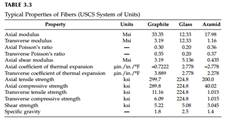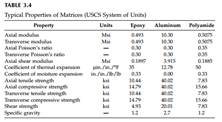### Create an Account

Already have account?

### Forgot Your Password ?

Home / Questions / Using elasticity model equations find the elastic moduli of a glass epoxy unidirectional ...

# Using elasticity model equations find the elastic moduli of a glass epoxy unidirectional lamina with 40% fiber volume fraction Use the properties of glass and epoxy from Table 33 and Table

Using elasticity model equations, find the elastic moduli of a glass/ epoxy unidirectional lamina with 40% fiber volume fraction. Use the properties of glass and epoxy from Table 3.3 and Table 3.4, respectively. Compare your results with those obtained by using the strength of materials approach and the Halphin–Tsai approach. Assume that the fibers are circularly shaped and are in a square array for the Halphin–Tsai approach.Jun 28 2020 View more View Less

#### Answer (Solved)Subscribe To Get Solution